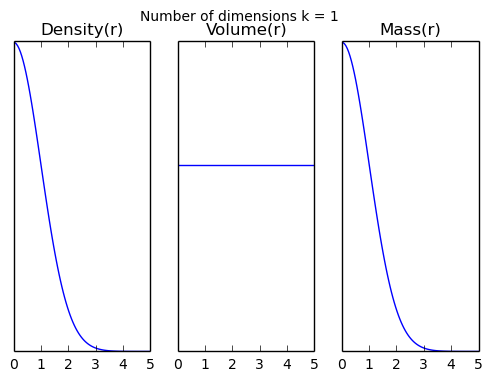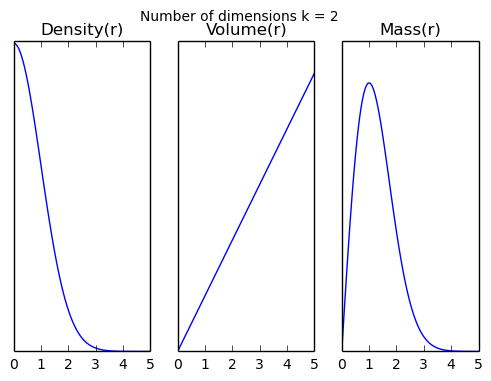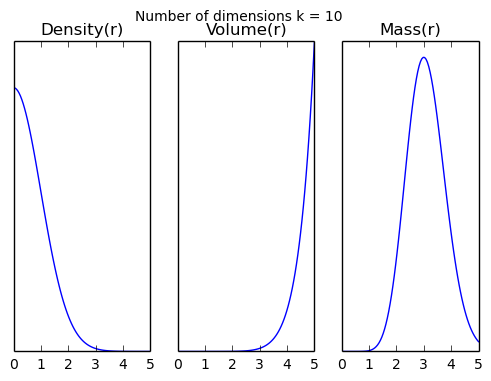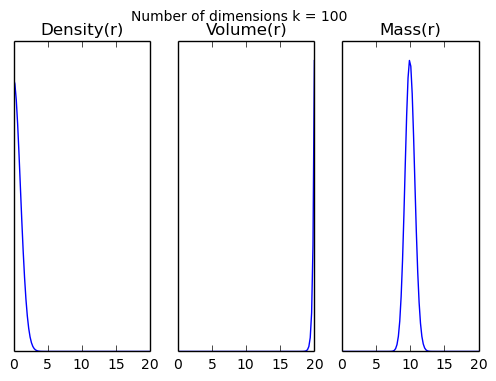# MCMC and the typical set of high dimensional random variables

21 March 2017

(This blog post was written as a Jupyter notebook. The .ipynb file of this blog post and associated files can be found here.)

I recently watched this talk by Michael Betancourt, and it helped to clarify for me some ideas about high dimensional distributions and sampling.

Specifically, I was aware of the fact that most of the probability mass of a (spherical) high-dimensional multivariate normal lies within a small distance of a hypersperical shell, with the probability mass concentrating closer and closer to the shell as the dimensionality increases. I was also vaguely aware that other high dimensional distributions I was dealing with had a similar property, that the majority of the probability mass lies close to some lower dimensional manifold, and that the proportion of the space that contains the majority of the probability mass becomes smaller and smaller as the dimensionality increases. But I'd missed the implication: that we can approximate any integral of a probability density function by integrating only over this lower dimensional manifold, if we can find it.

## Probability density, volume, and mass¶

As an aside, there are several ways to understand why the probability mass concentrates in this shell. Let's go back to the multivariate Gaussian case. In his talk, Betancourt explains that while, of course, the probability density is always greatest at the mode, and falls off faster as we move away from the mode as the dimensionality increases, the amount of volume in the space that is a given distance $r$ away from the mode increases with the dimensionality. The product of these two factors gives the amount of probability mass as a function of the distance from the mode $r$.

The following plots show the probability density, the amount of volume, and the probability mass (the product of the two) as a function of the distance from the mode $r$ for several values of the dimensionality $k$.

In :
import numpy as np
import matplotlib.pyplot as plt
%matplotlib inline
from scipy.special import gamma

In :
def probability_density_at_distance(r, k):
return (2*np.pi)**(-k/2.0) * np.exp(-0.5 * r**2)

def volume_at_distance(r, k):
return 2 * np.pi ** (k/2.0) * r**(k-1) / gamma(k/2.0)

v_probability_density_at_distance = np.vectorize(probability_density_at_distance)
v_volume_at_distance = np.vectorize(volume_at_distance)

def plot_density_volume_mass(k, max_r=5):
distances = np.linspace(0, max_r, 100)
densities = v_probability_density_at_distance(distances, k)
volumes = v_volume_at_distance(distances, k)
masses = densities * volumes

f, axarr = plt.subplots(1, 3)
f.suptitle("Number of dimensions k = %d" % k)
axarr.plot(distances, densities)
axarr.set_title("Density(r)")
axarr.plot(distances, volumes)
axarr.set_title("Volume(r)")
axarr.plot(distances, masses)
axarr.set_title("Mass(r)")
axarr.axes.get_yaxis().set_visible(False)
axarr.axes.get_yaxis().set_visible(False)
axarr.axes.get_yaxis().set_visible(False)

In :
plot_density_volume_mass(1)
plot_density_volume_mass(2)
plot_density_volume_mass(10)
plot_density_volume_mass(100, max_r=20)We can see that in 100 dimensions, the distance from the mean of a randomly sampled point from the standard normal distribution is much, much more likely to be 10 than any other value. The probability density out at $r=10$ is a factor of $5.2\times 10^{21}$ times smaller than the density at the mode, but in 100 dimensions there's just so much space out at that distance that it more than compensates for the difference.

## Relation to the sample standard deviation and the law of large numbers¶

The fact that most of the probability mass of a (spherical) high-dimensional multivariate normal lies within a thin hyperspherical shell can also be understood in terms of the fact that the sample standard deviation of a large sample from a normal distribution lies close to the population standard deviation.

Most people are comfortable with the idea that the standard deviation of a sample from a normal distribution gets closer to the scale parameter $\sigma$ as the sample size $N$ increases. Abusing notation slightly,

$$\lim_{N\to \infty} \sqrt{\frac{1}{N}\sum_{i=1}^{N}(x_i - \bar{x})^2} = \sigma\quad,$$

where $x_i$ is the $i$th sample. Interpreting $x_i$ to instead be the $i$th dimension of an $N$ dimensional Gaussian with covariance $\sigma \mathbb{1}_N$ (where $\mathbb{1}_N$ is the $N\times N$ identity matrix), and taking the $1/N$ outside of the square root we get

$$\lim_{N\to \infty} \sqrt{\sum_{i=1}^{N}(x_i - \bar{x})^2} = \sqrt{N}\sigma\quad.$$

In words, as the dimensionality increases, the distance of a sample from the mean will tend towards a particular value that depends on $N$.

## Why MCMC is hard in high dimensions¶

In Metropolis-Hastings MCMC, the next sample is determined by the following procedure:

1. Jump a random distance from the current sample. E.g., sample from a multivariate Gaussian centered on the current sample.
2. Accept or reject the new sample determined by the ratio of the probability density of the proposed new sample and the old sample.

As Betancourt says in his talk, this algorithm is nice and intuitive. In the first step, the probability of ending up in a given region of space is proportional to the volume of that space, and in the second step we account for the probability density.

But look again at the density—volume—mass plots for the multivariate Gaussian example above. In high dimensions, stepping in a random direction means—with overwhelming probability—stepping away from the mode. And that means that the ratio of the probability density at the proposed sample to the density at the current sample is going to be very small, so the sample will be rejected with very high probability.

The Hamiltonian Monte Carlo sampler used by Stan avoids this problem by using the gradient of the (log) probability density in step 1, i.e., in deciding where to hop to in the space.

## Integrating over the typical set of a Gaussian¶

I think it's cool that if you're integrating some function $f$ over a high dimensional spherical Gaussian, you can get a good approximation by just integrating over the surface of a hypersphere.

At first I thought that this idea might actually be useful, but then I realised that integrating over the surface of a high dimensional hypersphere is pretty fiddly.

Could we do it by sampling? I.e., sample $M$ points uniformly over the surface of the sphere and then compute $\frac{1}{M}\sum_{i=1}^{M}f(x_i)$.

It turns out it's not really worth it: the standard way to sample points uniformly over the surface of an $N$-sphere is to sample from an $N$ dimensional Gaussian and then project the points onto the sphere, and the whole idea here was to avoid sampling from the Gaussian! In any case, sampling from Gaussians is pretty easy.

In [ ]: